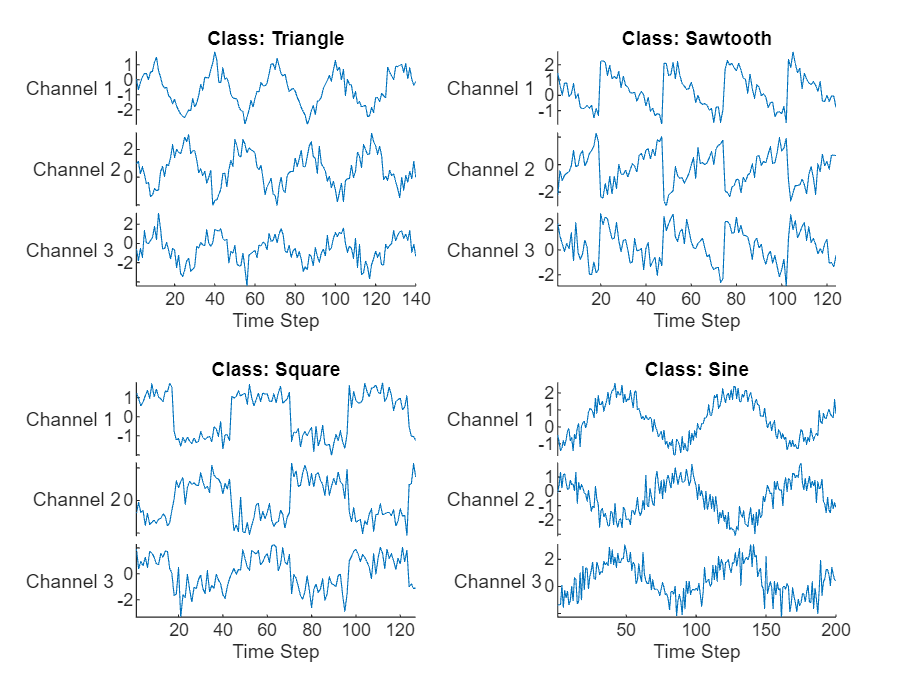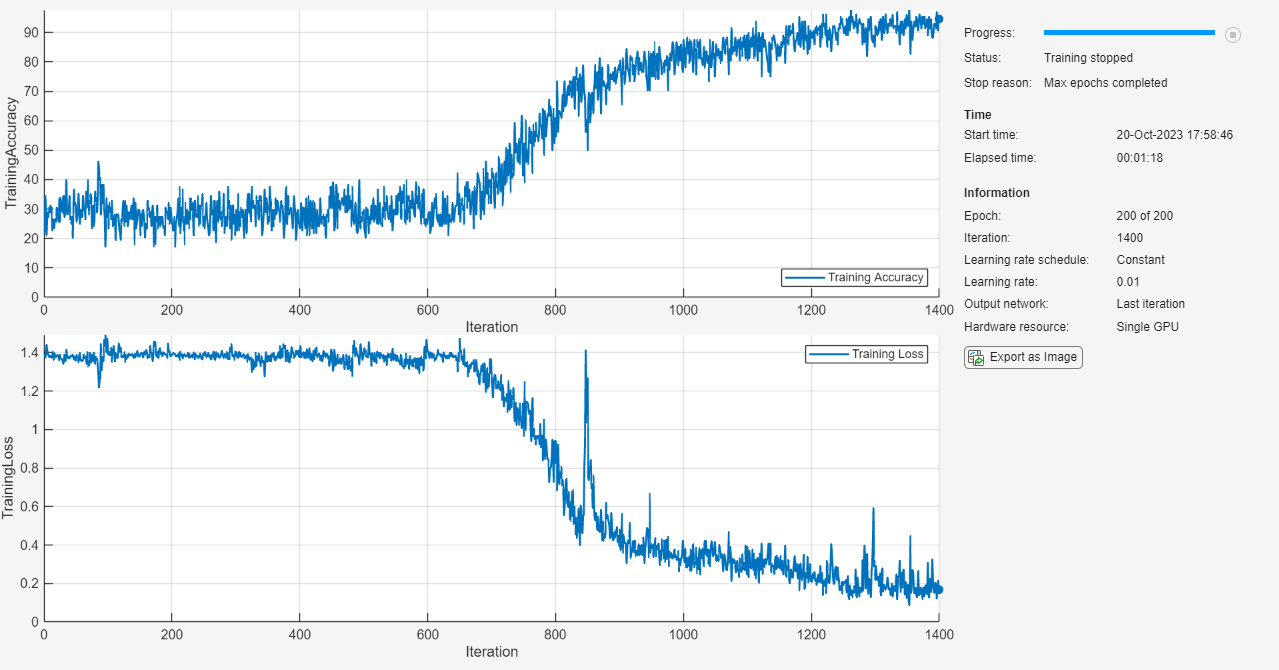Documentation

### This is machine translation

Mouseover text to see original. Click the button below to return to the English version of the page.

Note: This page has been translated by MathWorks. Click here to see
To view all translated materials including this page, select Country from the country navigator on the bottom of this page.

# sequenceInputLayer

Sequence input layer

## Description

A sequence input layer inputs sequence data to a network.

## Creation

### Syntax

``layer = sequenceInputLayer(inputSize)``
``layer = sequenceInputLayer(inputSize,Name,Value)``

### Description

````layer = sequenceInputLayer(inputSize)` creates a sequence input layer and sets the `InputSize` property.```

example

````layer = sequenceInputLayer(inputSize,Name,Value)` sets the optional `Normalization`, `Mean`, and `Name` properties using name-value pairs. You can specify multiple name-value pairs. Enclose each property name in single quotes.```

## Properties

expand all

### Image Input

Size of the input, specified as a positive integer or a vector of positive integers.

• For vector sequence input, `InputSize` is a scalar corresponding to the number of features.

• For 2-D image sequence input, `InputSize` is vector of three elements `[h w c]`, where `h` is the image height, `w` is the image width, and `c` is the number of channels of the image.

• For 3-D image sequence input, `InputSize` is vector of four elements `[h w d c]`, where `h` is the image height, `w` is the image width, `d` is the image depth, and `c` is the number of channels of the image.

Example: 100

Data transformation to apply every time data is forward propagated through the input layer, specified as one of the following.

• `'none'` — Do not transform the input data.

• `'zerocenter'` — Subtract the mean specified by the `Mean` property. The `trainNetwork` function automatically computes the mean at training time.

Mean used for zero center normalization, specified as a numeric array, or empty.

• For vector sequence input, `Mean` must be a `InputSize`-by-1 vector of means per channel.

• For 2-D image sequence input, `Mean` must be the same size as `InputSize` or be a 1-by-1-by-C array of means per channel, where C is the number of channels of the input. In this case, the number of channels of the input is `InputSize(3)`.

• For 3-D image sequence input, `Mean` must be the same size as `InputSize` or be a 1-by-1-by-1-by-C array of means per channel, where C is the number of channels of the input. In this case, the number of channels of the input is `InputSize(4)`.

You can set this property when creating networks without training (for example, when assembling networks using `assembleNetwork`). Otherwise, the `trainNetwork` function recomputes the mean at training time. When specifying the mean, you must also set the `Normalization` property to `'zerocenter'`.

Data Types: `single` | `double` | `int8` | `int16` | `int32` | `int64` | `uint8` | `uint16` | `uint32` | `uint64`

### Layer

Layer name, specified as a character vector or a string scalar. To include a layer in a layer graph, you must specify a nonempty unique layer name. If you train a series network with the layer and `Name` is set to `''`, then the software automatically assigns a name to the layer at training time.

Data Types: `char` | `string`

Number of inputs of the layer. The layer has no inputs.

Data Types: `double`

Input names of the layer. The layer has no inputs.

Data Types: `cell`

Number of outputs of the layer. This layer has a single output only.

Data Types: `double`

Output names of the layer. This layer has a single output only.

Data Types: `cell`

## Examples

collapse all

Create a sequence input layer with the name `'seq1'` and an input size of 12.

`layer = sequenceInputLayer(12,'Name','seq1')`
```layer = SequenceInputLayer with properties: Name: 'seq1' InputSize: 12 Hyperparameters Normalization: 'none' Mean: [] ```

Include an LSTM layer in a `Layer` array.

```inputSize = 12; numHiddenUnits = 100; numClasses = 9; layers = [ ... sequenceInputLayer(inputSize) lstmLayer(numHiddenUnits,'OutputMode','last') fullyConnectedLayer(numClasses) softmaxLayer classificationLayer]```
```layers = 5x1 Layer array with layers: 1 '' Sequence Input Sequence input with 12 dimensions 2 '' LSTM LSTM with 100 hidden units 3 '' Fully Connected 9 fully connected layer 4 '' Softmax softmax 5 '' Classification Output crossentropyex ```

Create a sequence input layer for sequences of 224-224 RGB images with the name `'seq1'`.

`layer = sequenceInputLayer([224 224 3], 'Name', 'seq1')`
```layer = SequenceInputLayer with properties: Name: 'seq1' InputSize: [224 224 3] Hyperparameters Normalization: 'none' Mean: [] ```

Train a deep learning LSTM network for sequence-to-label classification.

Load the Japanese Vowels data set as described in  and . `XTrain` is a cell array containing 270 sequences of varying length with a feature dimension of 12. `Y` is a categorical vector of labels 1,2,...,9. The entries in `XTrain` are matrices with 12 rows (one row for each feature) and a varying number of columns (one column for each time step).

`[XTrain,YTrain] = japaneseVowelsTrainData;`

Visualize the first time series in a plot. Each line corresponds to a feature.

```figure plot(XTrain{1}') title("Training Observation 1") numFeatures = size(XTrain{1},1); legend("Feature " + string(1:numFeatures),'Location','northeastoutside')```Define the LSTM network architecture. Specify the input size as 12 (the number of features of the input data). Specify an LSTM layer to have 100 hidden units and to output the last element of the sequence. Finally, specify nine classes by including a fully connected layer of size 9, followed by a softmax layer and a classification layer.

```inputSize = 12; numHiddenUnits = 100; numClasses = 9; layers = [ ... sequenceInputLayer(inputSize) lstmLayer(numHiddenUnits,'OutputMode','last') fullyConnectedLayer(numClasses) softmaxLayer classificationLayer]```
```layers = 5x1 Layer array with layers: 1 '' Sequence Input Sequence input with 12 dimensions 2 '' LSTM LSTM with 100 hidden units 3 '' Fully Connected 9 fully connected layer 4 '' Softmax softmax 5 '' Classification Output crossentropyex ```

Specify the training options. Specify the solver as `'adam'` and `'GradientThreshold'` as 1. Set the mini-batch size to 27 and set the maximum number of epochs to 100.

Because the mini-batches are small with short sequences, the CPU is better suited for training. Set `'ExecutionEnvironment'` to `'cpu'`. To train on a GPU, if available, set `'ExecutionEnvironment'` to `'auto'` (the default value).

```maxEpochs = 100; miniBatchSize = 27; options = trainingOptions('adam', ... 'ExecutionEnvironment','cpu', ... 'MaxEpochs',maxEpochs, ... 'MiniBatchSize',miniBatchSize, ... 'GradientThreshold',1, ... 'Verbose',false, ... 'Plots','training-progress');```

Train the LSTM network with the specified training options.

`net = trainNetwork(XTrain,YTrain,layers,options);`Load the test set and classify the sequences into speakers.

`[XTest,YTest] = japaneseVowelsTestData;`

Classify the test data. Specify the same mini-batch size used for training.

`YPred = classify(net,XTest,'MiniBatchSize',miniBatchSize);`

Calculate the classification accuracy of the predictions.

`acc = sum(YPred == YTest)./numel(YTest)`
```acc = 0.9297 ```

To create an LSTM network for sequence-to-label classification, create a layer array containing a sequence input layer, an LSTM layer, a fully connected layer, a softmax layer, and a classification output layer.

Set the size of the sequence input layer to the number of features of the input data. Set the size of the fully connected layer to the number of classes. You do not need to specify the sequence length.

For the LSTM layer, specify the number of hidden units and the output mode `'last'`.

```numFeatures = 12; numHiddenUnits = 100; numClasses = 9; layers = [ ... sequenceInputLayer(numFeatures) lstmLayer(numHiddenUnits,'OutputMode','last') fullyConnectedLayer(numClasses) softmaxLayer classificationLayer];```

For an example showing how to train an LSTM network for sequence-to-label classification and classify new data, see Sequence Classification Using Deep Learning.

To create an LSTM network for sequence-to-sequence classification, use the same architecture as for sequence-to-label classification, but set the output mode of the LSTM layer to `'sequence'`.

```numFeatures = 12; numHiddenUnits = 100; numClasses = 9; layers = [ ... sequenceInputLayer(numFeatures) lstmLayer(numHiddenUnits,'OutputMode','sequence') fullyConnectedLayer(numClasses) softmaxLayer classificationLayer];```

To create an LSTM network for sequence-to-one regression, create a layer array containing a sequence input layer, an LSTM layer, a fully connected layer, and a regression output layer.

Set the size of the sequence input layer to the number of features of the input data. Set the size of the fully connected layer to the number of responses. You do not need to specify the sequence length.

For the LSTM layer, specify the number of hidden units and the output mode `'last'`.

```numFeatures = 12; numHiddenUnits = 125; numResponses = 1; layers = [ ... sequenceInputLayer(numFeatures) lstmLayer(numHiddenUnits,'OutputMode','last') fullyConnectedLayer(numResponses) regressionLayer];```

To create an LSTM network for sequence-to-sequence regression, use the same architecture as for sequence-to-one regression, but set the output mode of the LSTM layer to `'sequence'`.

```numFeatures = 12; numHiddenUnits = 125; numResponses = 1; layers = [ ... sequenceInputLayer(numFeatures) lstmLayer(numHiddenUnits,'OutputMode','sequence') fullyConnectedLayer(numResponses) regressionLayer];```

For an example showing how to train an LSTM network for sequence-to-sequence regression and predict on new data, see Sequence-to-Sequence Regression Using Deep Learning.

You can make LSTM networks deeper by inserting extra LSTM layers with the output mode `'sequence'` before the LSTM layer. To prevent overfitting, you can insert dropout layers after the LSTM layers.

For sequence-to-label classification networks, the output mode of the last LSTM layer must be `'last'`.

```numFeatures = 12; numHiddenUnits1 = 125; numHiddenUnits2 = 100; numClasses = 9; layers = [ ... sequenceInputLayer(numFeatures) lstmLayer(numHiddenUnits1,'OutputMode','sequence') dropoutLayer(0.2) lstmLayer(numHiddenUnits2,'OutputMode','last') dropoutLayer(0.2) fullyConnectedLayer(numClasses) softmaxLayer classificationLayer];```

For sequence-to-sequence classification networks, the output mode of the last LSTM layer must be `'sequence'`.

```numFeatures = 12; numHiddenUnits1 = 125; numHiddenUnits2 = 100; numClasses = 9; layers = [ ... sequenceInputLayer(numFeatures) lstmLayer(numHiddenUnits1,'OutputMode','sequence') dropoutLayer(0.2) lstmLayer(numHiddenUnits2,'OutputMode','sequence') dropoutLayer(0.2) fullyConnectedLayer(numClasses) softmaxLayer classificationLayer];```

Create a deep learning network for data containing sequences of images, such as video and medical image data.

• To input sequences of images into a network, use a sequence input layer.

• To apply convolutional operations independently to each time step, first convert the sequences of images to an array of images using a sequence folding layer.

• To restore the sequence structure after performing these operations, convert this array of images back to image sequences using a sequence unfolding layer.

• To convert images to feature vectors, use a flatten layer.

You can then input vector sequences into LSTM and BiLSTM layers.

Define Network Architecture

Create a classification LSTM network that classifies sequences of 28-by-28 grayscale images into 10 classes.

Define the following network architecture:

• A sequence input layer with an input size of `[28 28 1]`.

• A convolution, batch normalization, and ReLU layer block with 20 5-by-5 filters.

• An LSTM layer with 200 hidden units that outputs the last time step only.

• A fully connected layer of size 10 (the number of classes) followed by a softmax layer and a classification layer.

To perform the convolutional operations on each time step independently, include a sequence folding layer before the convolutional layers. LSTM layers expect vector sequence input. To restore the sequence structure and reshape the output of the convolutional layers to sequences of feature vectors, insert a sequence unfolding layer and a flatten layer between the convolutional layers and the LSTM layer.

```inputSize = [28 28 1]; filterSize = 5; numFilters = 20; numHiddenUnits = 200; numClasses = 10; layers = [ ... sequenceInputLayer(inputSize,'Name','input') sequenceFoldingLayer('Name','fold') convolution2dLayer(filterSize,numFilters,'Name','conv') batchNormalizationLayer('Name','bn') reluLayer('Name','relu') sequenceUnfoldingLayer('Name','unfold') flattenLayer('Name','flatten') lstmLayer(numHiddenUnits,'OutputMode','last','Name','lstm') fullyConnectedLayer(numClasses, 'Name','fc') softmaxLayer('Name','softmax') classificationLayer('Name','classification')];```

Convert the layers to a layer graph and connect the `miniBatchSize` output of the sequence folding layer to the corresponding input of the sequence unfolding layer.

```lgraph = layerGraph(layers); lgraph = connectLayers(lgraph,'fold/miniBatchSize','unfold/miniBatchSize');```

View the final network architecture using the `plot` function.

```figure plot(lgraph)```M. Kudo, J. Toyama, and M. Shimbo. "Multidimensional Curve Classification Using Passing-Through Regions." Pattern Recognition Letters. Vol. 20, No. 11–13, pages 1103–1111.

 UCI Machine Learning Repository: Japanese Vowels Dataset. https://archive.ics.uci.edu/ml/datasets/Japanese+Vowels

Download ebook